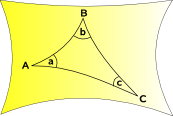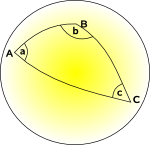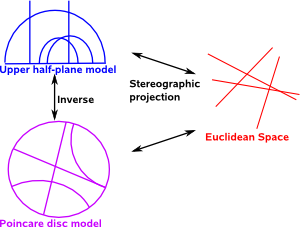# Maths - Hyperbolic Geometry

In Rienmannian geometry space can curve at different places (see manifolds) here we look at geometries where the curve of space is constant.

 Eulidean Geometry flat spaceHyperbolic Geometry space curves outwardSpherical Geometry and Elliptic Geometry space curves inward## Terminology

Here we look at the terminology such as geometries, spaces, models, projections and transforms. Its quite difficult when we start dealing with non-Euclidean geometries because we use similar terminology that we are used to in conventional Euclidean space but the terms can have slightly different properties. For example, the concept of a 'line' can look different in different geometries:### Geometries and Spaces

Rienmannian geometry defines spaces generally in terms of manifolds, here we are interested in homogeneous, isotropic spaces which have no preferred points or directions, examples are:

parallel postulate - number of unique parallel lines through point space curves Inventors Point at Infinity Distance Measure
Eulidean Geometry 1 none Euclid where parallel lines meet √(x² + y²)
Hyperbolic Geometry space curves outward so lines dont meet Lobachevskii
and Bolyai

Spherical Geometry

Elliptic Geometry

0 space curves inward so all lines meet

Examples are:

### Transforms

Invariant

Examples are:

• Möbius Transform
• Lorentz Transform

## Parallel Postulate

If we take away the parallel postulate from Euclidean Space.

• parallel postulate is false
• the angles of a triangle do not add to π
• for a shape of a given size, there does not in general, exist a similar shape of a larger size.

Possible mappings between Euclidean Space and Hyperbolic Space:

properties:

projective conformal (Poincaré disc )
straight lines - geodesics   segments of circles
angle   preserved
internal angles of triangle   π-(α+β+γ)=CΔ
distance between two points   lnGeometric Algebra for Computer Science: An Object-oriented Approach to Geometry. This book stresses the Geometry in Geometric Algebra, although it is still very mathematically orientated. Programmers using this book will need to have a lot of mathematical knowledge. Its good to have a Geometric Algebra book aimed at computer scientists rather than physicists. There is more information about this book here.